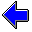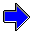Java Security Settings:
This web page employs Java, which requires specific security settings for correct operation.
If the applets on this page do not run correctly, consult the Virtual Chemistry Experiments FAQ
or the Physlet Physics web site for establishing the correct security settings.

# Chemical Kinetics

## Isolation Method

### Concepts

A common challenge in chemical kinetics is to determine the rate law for a reaction with multiple reactants. With the concentrations of several species changing simultaneously, real rate laws often do not follow the simple zero-, first-, and second-order rate laws previously discussed. The Method of Initial Rates can, in principle, be used to determine the rate law, but in practice the Method of Initial Rates poses serious experimental difficulties. The primary problem is the difficulty in accurately determining the initial rate.

A better strategy is the Isolation Method. In this method the concentration of one reactant is made much smaller than the concentrations of the other reactants. Under this condition, all reactant concentrations except one are essentially constant, and the simple zero-, first-, and second-order kinetic plots can usually be used to interpret the concentration-time data.

To see how this method works, consider the following substitution reaction:

CH3Br (aq) + OH- (aq)   →   CH3OH (aq) + Br- (aq)

The rate law for this substitution reaction is first-order in bromomethane and first-order in hydroxide ion, second-order overall.

r = k [CH3Br] [OH-]

Suppose the reaction were performed starting with 0.100 mole/L OH- and 0.00100 mole/L CH3Br. When the reaction is finished, the solution will contain 0.099 mole/L OH- and essentially no CH3Br. Notice that the concentration of hydroxide ion is essential unchanged. (The total change is 1%, which is often comparable to or smaller than the experimental error.) The value of [OH-] can therefore be treated as a constant, and the rate law becomes

r = kobs [CH3Br]

which is a simple first-order rate law. The observed rate constant, kobs, is a pseudo-first-order rate constant. The reaction is a pseudo-first-order reaction, because it behaves as if it were first-order though it is not really first-order. The difference is that kobs is not a true constant; its value varies with the initial concentration of hydroxide ion.

kobs = k [OH-]

In practice, one would perform a series of experiments in which [OH-] varies but was always much larger than the initial [CH3Br]. For each experiment, kobs would be determined, and one would then prepare a plot of kobs vs [OH-]. Such a plot should be linear with a slope of k.

If one did not know the reaction was first-order in hydroxide ion, the exponent for [OH-] could also be determined from the experimental data. The observed rate constant would be

kobs = k [OH-]b

Taking the logarithm of both sides of this equation leads to

ln kobs = ln k + b ln [OH-]

A plot of ln kobs vs ln [OH-] produces a straight line with slope = b and intercept = ln k. Thus one can simultaneously determine b and k, and the method works for any value of b.

How large a concentration excess is required to effectively "isolate" the effects of a single reactant? As a general rule, a minimum of a 20-fold stoichiometric excess is necessary. A 50-fold or 100-fold stoichiometric excess is preferrable.

### Experiment

Objectives

• Determine the rate law for a chemical reaction.
• Determine the rate constant for a chemical reaction.

An important reaction that occurs in the atmosphere is

2 NO (g) + O2 (g)   →   2 NO2 (g)

Nitrogen oxides (NOx) are produced by automobile engines, and the fate of these oxides in the environment is of considerable concern. Modeling reactions involving nitric oxide (NO) and nitrogen dioxide (NO2) are important in understanding the behavior of air pollution and in developing effective regulations. Nitric oxide is a very reactive, colorless gas; nitrogen dioxide is an orange-brown gas that is responsible for the characteristic color of Los Angeles smog.

In this experiment, the stopped-flow apparatus has been adapted to handle gases. Both syringes are charged with gas mixtures at 1 atm pressure. The syringe on the right contains 99% N2 and 1% NO, which at 25o C corresponds with 4.09 x 10-4 mole/L NO. The syringe on the left contains pure oxygen, which corresponds to 0.0409 mole/L O2. Both syringes are loaded with 10 mL of gas and at least 1 mL must be discharged during an experiment.

The reactants in the reaction are colorless; the NO2 product has an orange-brown color, and it is thus the NO2 concentration that is measured experimentally. The graph at the right shows how the concentration of NO2 varies with time (the characteristic zero-order kinetic plot). By clicking the appropriate button, characteristic first-order and second-order kinetic plots can also be prepared. (In these cases, it is necessary to plot [NO2]f - [NO2] rather than [NO2], because NO2 is a product rather than a limiting reactant. [NO2]f is the final nitrogen dioxide concentration, which is known from the initial concentrations and the reaction stoichiometry.

Perform this reaction several times, taking care to make sure that oxygen is always present in large excess. Use the characteristic kinetic plot to determine the order of the reaction with respect to NO and the pseudo-rate constant for the chosen oxygen concentration. (Remember to take into account the reaction stoichiometry when calculating the rate constant.)

For each experiment, enter the oxygen concentration and the pseudo-rate constant in the table at the right. The points in the table are used to construct a ln kobs vs ln [O2] plot in the graph at the left. Use the slope and intercept of this plot to determine the order of the reaction with respect to oxygen and the reaction rate constant.

There is experimental uncertainty in the absorbance, and this random error will introduce scatter in the kinetics plots, especially when the concentration of nitrogen dioxide (the light-absorbing species) is small. If possible, try to avoid using conditions that produce only small amount of nitrogen dioxide. The error will also produce extreme scattering in a kinetic plot when the NO2 concentration produces the final value. Such plots should only use data over the first three or four half-lives of the reaction; though in this experiment you will not have control over the reaction time.

Volumes Delivered

Oxygen:   mL
Nitric Oxide:   mL

Kinetic Plots

C vs t
ln[Cf - C] vs t
1/(Cf - C) vs t

slope:
intercept:
Data Points
ln [O2] ln kobs

Oxygen Concentration
mole/L

Observed Rate Constant

slope

interceptMethod of Initial Rates                     Kinetics of the Bromate-Bromide ReactionChemical Kinetics Home PageVirtual Chemistry Home Page

IsolationMethod.html version 2.1
© 2000-2014 David N. Blauch